GotW #74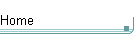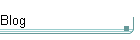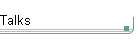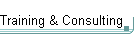On theblogNovember 4: Other Concurrency Sessions at PDC November 3 October 26: Hoare on Testing October 23This is the original GotW problem and solution substantially as posted to Usenet. See the book Exceptional C++ Style (Addison-Wesley, 2004) for the most current solution to this GotW issue. The solutions in the book have been revised and expanded since their initial appearance in GotW. The book versions also incorporate corrections, new material, and conformance to the final ANSI/ISO C++ standard (1998) and its Technical Corrigendum (2003).

## Uses and Abuses of Vector Difficulty: 4 / 10

Almost everybody uses `std::vector`, and that's good. Unfortunately, many people misunderstand some of its semantics and end up unwittingly using it in surprising and dangerous ways. How many of the subtle problems illustrated in this issue might be lurking in your current program?### Problem

#### JG Question

1. Given a `vector<int> v`, what is the difference between the lines marked A and B?

``````// Example 1: [] vs. at()
//
void f( vector<int>& v )
{
v;      // A
v.at(0);   // B
}``````

#### Guru Question

2. Consider the following code:

``````// Example 2: Some fun with vectors
//
vector<int> v;

v.reserve( 2 );
assert( v.capacity() == 2 );
v = 1;
v = 2;
for( vector<int>::iterator i = v.begin();
i < v.end(); i++ )
{
cout << *i << endl;
}

cout << v;
v.reserve( 100 );
assert( v.capacity() == 100 );
cout << v;

v = 3;
v = 4;
// ...
v = 100;
for( vector<int>::iterator i = v.begin();
i < v.end(); i++ )
{
cout << *i << endl;
}``````

Critique this code. Consider both style and correctness.### Solution

#### Accessing Vector Elements

1. Given a `vector<int> v`, what is the difference between the lines marked A and B?

``````// Example 1: [] vs. at()
//
void f( vector<int>& v )
{
v;      // A
v.at(0);   // B
}``````

In Example 1, if `v` is not empty then there is no difference between lines A and B. If `v` is empty, then line B is guaranteed to throw a `std::out_of_range` exception, but there's no telling what line A might do.

There are two ways to access contained elements within a `vector`. The first, `vector<T>::at()`, is required to perform bounds checking to ensure that the `vector` actually contains the requested element. It doesn't make sense to ask for, say, the 100th element in a `vector` that only contains 10 elements at the moment, and if you try to do such a thing, `at()` will protest by throwing a `std::out_of_range` hissy fit (a.k.a. "exception").

On the other hand, `vector<T>::operator[]()` is allowed, but not required, to perform bounds checking. There's not a breath of wording in the standard's specification for `operator[]()` that says anything about bounds checking, but neither is there any requirement that it have an exception specification, so your standard library implementer is free to add bounds-checking to `operator[]()`, too. So, if you use `operator[]()` to ask for an element that's not in the `vector`, you're on your own, and the standard makes no guarantees about what will happen (although your standard library implementation's documentation might) -- your program may crash immediately, the call to `operator[]()` might throw an exception, or things may seem to work and occasionally and/or mysteriously fail.

Given that bounds checking protects us against many common problems, why isn't `operator[]()` required to perform bounds checking? The short answer is: Efficiency. Always checking bounds would cause a (possibly slight) performance overhead on all programs, even ones that never violate bounds. The spirit of C++ includes the dictum that, by and large, you shouldn't have to pay for what you don't use, and so bounds checking isn't required for `operator[]()`. In this case we have an additional reason to want the efficiency: `vector`s are intended to be used instead of built-in arrays, and so should be as efficient as built-in arrays, which don't do bounds-checking. If you want to be sure that bounds get checked, use `at()` instead.

#### Size()ing Up Vector

Let's turn now to Example 2, which manipulates a `vector<int>` using a few simple operations.

2. Consider the following code:

``````// Example 2: Some fun with vectors
//
vector<int> v;

v.reserve( 2 );
assert( v.capacity() == 2 );``````

This assertion has two problems, one substantive and one stylistic.

1. The substantive problem is that this assertion might fail.

Why might it fail? Because the call to `reserve()` will guarantee that the vector's `capacity()` is at least 2 -- but it might also be greater than 2, and indeed is likely to be greater because a `vector`'s size must grow exponentially and so typical implementations may choose to always grow the internal buffer on exponentially increasing boundaries even when specific sizes are requested via `reserve()`.

So this comparison should instead test using `>=`, not strict equality:

``assert( v.capacity() >= 2 );``

This leaves us with the other potential issue:

2. The stylistic problem is that the (corrected) assertion is redundant.

The standard already guarantees what is here being asserted. Why add needless clutter by testing for it explicitly? This doesn't make sense unless you suspect a bug in the standard library implementation you're using, in which case you have bigger problems.

``````
v = 1;
v = 2;``````

Both of the above lines are flat-out errors, but they might be hard-to-find flat-out errors because they'll likely "work" after a fashion on your implementation of the standard library.

There's a big difference between `size()` (which goes with `resize()`) and `capacity()` (which goes with `reserve()`):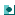`size()` tells you how many elements are currently actually present in the container, and `resize()` adjusts the actual contents of the container to be the specified size by adding or removing elements at the end. Both functions are available for `list`, `vector`, and `deque`, not other containers.`capacity()` tells you how many elements have room before adding another would force the `vector` to allocate more space, and `reserve()` grows (never shrinks) into a larger internal buffer if necessary to ensure at least the specified space is available. Both functions are available only for `vector`.

In this case, we used `v.reserve(2)` and therefore know that `v.capacity() >= 2`, and that's fine as far as it goes -- but we never actually added any elements to `v`, so `v` is still empty! At this point `v` just happens to have room for two or more elements.

We can only safely use `operator[]()` (or `at()`) to modify elements that are actually present, which means that they count towards `size()`. At first you might wonder why `operator[]()` couldn't just be smart enough to add the element if it's not already there, but if `operator[]()` were allowed to work this way, you could create a `vector` with "holes" in it! For example, consider:

`vector<int> v;v.reserve( 100 );v = 42; // if this were allowed, then...// ...now what are the values of v[0..98]?`

Alas, because `operator[]()` isn't required to perform range checking, on most implementations the expression "`v`" will simply return a reference to the not-yet-used space in the `vector`'s internal buffer where the first element would eventually go. Therefore, the statement "`v = 1;`" will probably appear to work, kind of, sort of, in that if the program were to `cout << v` now the result would probably be 1, just as (mis)expected. Again, whether this will actually happen on the implementation you're using isn't guaranteed; it's just one typical possibility. The standard puts no requirements on what the implementation should do with flat-out errors like writing "`v`" for an empty vector `v`, because the programmer is assumed to know enough not to write such things -- and after all, if the programmer had wanted the library to perform bounds checking, he would have written "`v.at(0)`", right?

Of course, the assignments "`v = 1; v = 2;`" would have been fine if the earlier code had performed a `v.resize(2)` instead of just a `v.reserve(2)` -- but it didn't, so they're not. Alternatively, it would have been fine to replace them with "`v.push_back(1); v.push_back(2);`" which is the always-safe way to tack elements onto the end of a container.

`for( vector<int>::iterator i = v.begin();      i < v.end(); i++ ){  cout << *i << endl;}`

First, note that this loop prints nothing because of course the `vector` is still empty. This might surprise the original programmer, until that programmer realizes that the above code never really added anything to the `vector` -- it was just (dangerously) playing around with some of the reserved but still-officially-unused space hanging around inside the `vector`.

Having said that, there is no outright error in the above loop, but there are several stylistic problems that I would comment on if I saw this code in a code review setting. Most are low-level comments:

1. Be as const-correct as possible.

The iterator is never used to modify the `vector`'s contents, so it should be a `const_iterator`.

2. Prefer comparing iterators with `!=`, not `<`.

True, because `vector<int>::iterator` happens to be a random-access iterator (not necessarily an `int*`, of course!), there's no downside to using `<` in the comparison with `v.end()` above. But `<` only works with random-access iterators, whereas `!=` works with other iterator types too, so we should routinely use `!=` all the time unless we really need `<`.

3. Prefer using prefix `--` and `++`, instead of postfix.

Get in the habit of by default writing `++i` instead of `i++` in loops unless you really need the old value of `i`. For example, postfix is natural and fine when you're writing something like "`v[i++]`" to access the `i`-th element and at the same time increment a loop counter.

In this case, there's almost certainly no performance difference because often `vector<int>::iterator` really is just a plain old `int*` which is cheap to copy and anyway can have extra copies optimized away by the compiler, because the compiler is allowed to know about the semantics of `int*`'s.

4. Avoid needless recalculations.

In this case, `v.end()` doesn't change during the loop, so instead of recalculating it on every iteration it might be worthwhile to precalculate it before the loop begins.

Note: If your implementation's `vector<int>::iterator` is just an `int*`, and your implementation inlines `end()` and does reasonable optimization, it's probable that there's zero overhead here anyway because the compiler will probably be able to see that the value of `end()` doesn't change and that it can therefore safely hoist the code out of the loop. This is a pretty common case. However, if your implementation's `vector<int>::iterator` is not an `int*` (for example, if it's a debugging implementation it could be of class type), the function(s) are not inlined, and/or the compiler doesn't perform the suggested optimizations, then hoisting the calculation code out of the loop yourself can make a performance difference.

5. Prefer `'\n'` to `endl`.

Using `endl` forces the stream to flush its internal output buffers. If the stream is buffered and you don't really need a flush each time, just write a flush once at the end of the loop and your program will perform that much faster.

Finally, the last comment hits at a higher level:

6. Prefer reusing the standard library's `copy()` and `for_each()` instead of handcrafting your own loops, where using the standard facilities is clean and easy. Season to taste.

I say "season to taste" because here's one of those places where taste really does matter. In simple cases, `copy()` and `for_each()` really can and do improve readability over handcrafted loops. Beyond those simple cases, though, unless you have a nice expression template library available, code written using `for_each()` can get unreadable pretty quickly because the code in the loop body has to be split off into functors. Sometimes that kind of factoring is still a good thing; other times it's merely obscure.

That's why your tastes may vary. Still, in this case I would be tempted to replace the above loop with something like:

`copy( v.begin(), v.end(),      ostream_iterator<int>(cout, "\n") );`

Besides, when you reuse `copy()` like this, you can't get the `!=`, `++`, `end()`, and `endl` parts wrong because they're done for you. (Of course, this assumes that you don't want to flush the output stream after each `int` is output; if you do, there's no way to do it without writing your own loop instead of reusing `std::copy()`.) Reuse, when applied well, not only makes code more readable but can also make it better by avoiding some opportunities for pitfalls.

You can take it a step further and write a container-based algorithm for copying -- that is, an algorithm that operates on an entire container, not just an iterator range. Doing this would also automatically get the `const_iterator` part right. For example:

`template<class Container, class OutputIterator>OutputIterator copy( const Container& c,`                    ` OutputIterator result ){  return std::copy( c.begin(), c.end(), result );}`

Here we simply wrap `std::copy()` for an entire container, and because the container is taken by `const&` the iterators will automatically be `const_iterator`s.

`cout << v;`

When the program performs "`cout << v`" now, it will probably produce a 1. This is because the program scribbled over memory in a way that it shouldn't have, but that will probably not cause an immediate fault -- more's the pity.

`v.reserve( 100 );assert( v.capacity() == 100 );`

Again, this assertion should use `>=`, and then becomes redundant anyway, as before.

`cout << v;`

Surprise! This time, the "`cout << v`" will probably produce a 0 -- the value 1 that we just set has mysteriously vanished!

Why? Assuming that the `reserve(100)` actually did trigger a reallocation of `v`'s internal buffer (i.e., if the initial call to `reserve(2)` didn't already raise `capacity()` to 100 or more), `v` would only copy over into the new buffer the elements it actually contains -- and it doesn't actually think it contains any! The new buffer initially holds zeroes, and that's what stays there.

`v = 3;v = 4;// ...v = 100;`

No doubt you are even now shaking your head sadly at this deplorable code. This is bad, bad, bad... but because `operator[]()` isn't required to perform bounds-checking, on most implementations this will probably silently appear to work and won't cause an immediate exception or memory trap.

`v.at(2) = 3;v.at(3) = 4;// ...v.at(99) = 100;`

then the problem would have become obvious, because the very first call would have thrown an `out_of_range` exception.

`for( vector<int>::iterator i = v.begin();     i < v.end(); i++ ){  cout << *i << endl;}`

Again this prints nothing, and I'd consider replacing it with:

`copy( v.begin(), v.end(),       ostream_iterator<int>(cout, "\n") );`

Notice again that this reuse automatically solves the `!=`, prefix `++`, `end()`, and `endl` comments too -- the opportunity to get them wrong never arises! Good reuse often makes code automatically faster and safer, too.

#### Summary

Know the difference between `size()` and `capacity()`. Know also the difference between `operator[]()` and `at()`, and always use the latter if you want bounds-checked access. Doing so can easily save you long hours of sweat and tears inside a debugger.